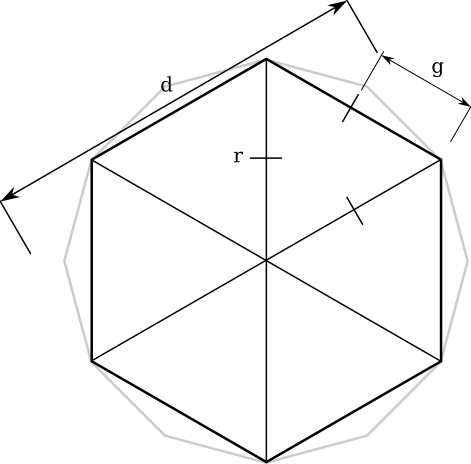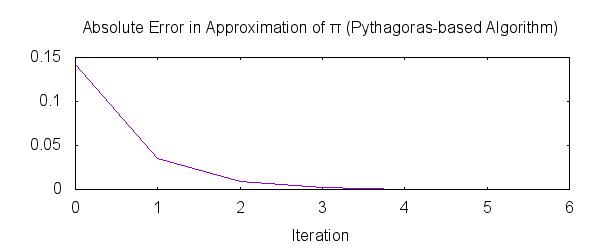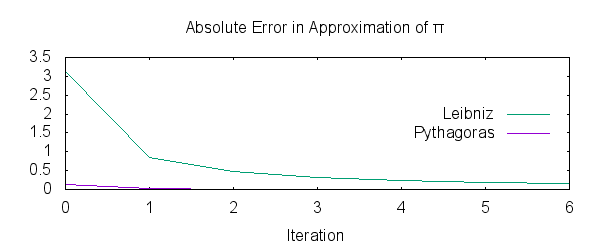Main categories:

# Pi-Thagoras

If pi is the ratio between a circle's circumference and its diameter, then a circle with a diameter of 1 unit will have a circumference equal to pi units. If a circle is nothing but a regular polygon with an infinite number of sides, then we can surely approximate pi using a polygon with a very large number of sides.

This page is a summary of work and thought I put in over a period roughly spanning mid 2014 through early 2015.

## A Simple Theory

Before I continue, I would just like to inb4 that I know this can be calculated using methods vastly more efficient and countless times less "round-about". I chose to pursue this for the pure academic challenge.

Using some simple algebra, I came up with the below expression for the length of one side of a 2k-gon given the length of a side on an k-gon:

gn+1 = 0.5 √(0.5 − √(0.25 − gn2))

Of course, we can find the perimeter of an n-gon by summing up all its side lengths. Since we are using regular polygons, this is as easy as multiplying the length of one side by the total number of sides.

To recap so far: We have a method to get finer and finer polygons with more and more detail, in order to approach a polygon of infinite sides with perimeter π. To bridge the gap between "all this theory sure is great" and "holy crap, that's pi," we need a starting point though; a polygon with known radius and known side length. Possibly the simplest solution lies in the equilateral triangle. If we build a hexagon out of these, we have a polygon with a tidy radius 0.5 (remember it's nice to have the diameter set to 1), and therefore a side length of 0.5 units too.From here, we can iterate the expression over and over, calculating a 12-gon's geometry, followed by a 24-gon, followed by 48-, 96-, 192-, 384-, 768-gons and so on. Each time, we will have a polygon approaching an ∞-gon (a circle), and therefore a perimeter approaching pi.

## A Simple Implementation

Let's implement this very quickly in C.

``````#include <stdio.h>
#include <math.h>

int main()
{
/* initial (hexagon) values */
long sides = 6;
double side_len = 0.5;

double pi = 0;
double last_pi = 0;

do
{
last_pi = pi;
side_len = sqrt(0.5 - sqrt(0.25 - pow(side_len/2, 2)));
sides *= 2;
pi = side_len * sides;
printf("%.60f\n", pi);
} while(pi != last_pi);
}
``````

The best pi I can squeeze out of that is (with incorrect digits ruled out) 3.141592645321215737652664756751619279384613037109375. Hmm. Eight digits. That's pretty poor, really. Well, in the big scheme of things, sure, it's alright. It's π±10-7. Even in modelling simple physical systems, all you need is 3.14, maybe 3.142 if it's a Thursday.

Looking at the algorithm's absolute error against the iteration number shows that it's has an asymptotic nature:I decided to plot the absolute error in an algorithm calculating one term of the Leibniz series per iteration. The result was quite surprising to me:Wow. This Pythagoras-based thing isn't the worst thing there is.

It is very easy to get the green plot on the lower graph confused with the purple one on the upper graph, but these are not the same data sets.

The graphs don't show it, but it takes 8 iterations before the Leibniz-based algorithm gets its absolute error lower than the Pythagoras-based algorithm's absolute error at its 0th iteration. With that small confidence boost, let's calculate more precision than humans will actually ever need (for the umpteenth time in history).

## A Less Simple Implementation

So far, we have identified one main area that we should improve upon: our precision is lacking. The solution? Arbitrary precision. Crank it up high.

Despite the fact that it sucks, I opted to use GMP as my arbitrary precision library. In essence, it will let me use, say 8 megabit floats rather than silly little 64 bit ones. I wrote up a quick implementation of the algorithm using GMP and gave it a spin. First, I asked it to calculate 1000 digits for me and it gave just over 1000 correct digits. Later, I asked it to calculate the first 1 000 000 digits of pi and (a few days later) it got 999 999 correct. Fixing minor problems like this are out of the scope of this experiment, which means I get to be lazy.

I won't put the entirety of the code on this web page because of its signal-to-noise ratio (about ⅘ of its lines are swarf). Here's the core component, if you really must:

``````    while (mpf_cmp(oldpi, pi) != 0)
{
mpf_set(oldpi, pi);
mpf_div_ui(length, full_length, 2);
mpf_mul(length, length, length);

mpf_sqrt(temp, temp);
mpf_sqrt(full_length, temp);

mpf_mul_ui(sides,sides,2);
mpf_mul(pi, full_length, sides);
}
``````

It's a pain to read without documentation, so here are a few key notes:

• `length` is equivalent to gn
• `radius` is the constant, 0.5
• `radius_2` is the constant (equal to `radius` squared) 0.25

## Footnotes

1. I know that Leibniz series is just that—a series, and not at all an algorithm for pi.
2. Suckless says GMP sucks but at the time of writing, the only alternatives they offer don't support floating point.